## Different Types of Unsupervised Machine Learning Algorithms

Posted on 2020-12-11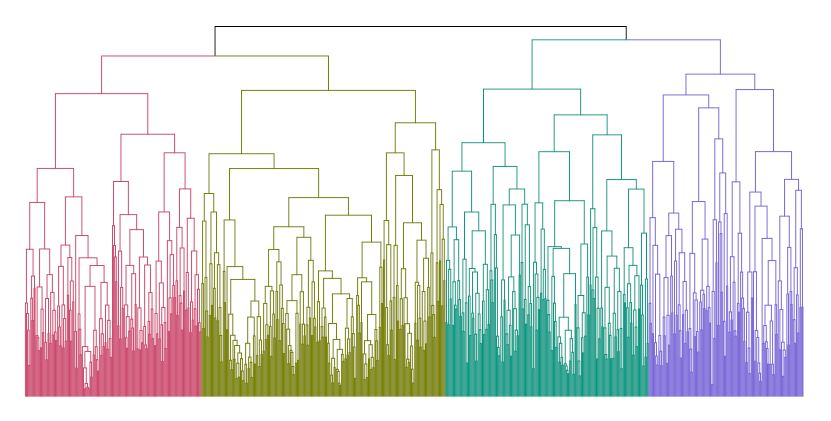An unsupervised machine learning model handles unlabeled dataset that the algorithm tries to make sense by extracting features and patterns on its own. In other words, its main purpose is exploration. It tries to explore the structure of the data and organize similar data points into groups or clusters. In order to make that happen, unsupervised learning applies one major technique: clustering.

What is clustering?

Clustering involves assigning data points to clusters such that the data points that belong to a cluster are similar. At the same time, clusters generated are dissimilar or different from other clusters. There are several ways to generate clusters, which have been organized into a few distinct categories: Partitional, Hierarchical, and Bayesian.

Partitional Clustering

Partitional clustering organizes data into non-hierarchical clusters that do not overlap. As such, it includes the following algorithms:

1.      K-means

In this approach, it requires the user to specify the number of clusters (K). It then randomly selects K distinct data points as centroids, and each data point are assigned to a cluster belonging to the closest centroid. It then measures the distance of all data points from all centroids, and re-assigns the centroids based on the cluster means. The steps are repeated until the cluster centroids no longer move.

However, there are some limitations to this algorithm. It is sensitive to outliers and does not cluster well whenever the data are not arranged in a circular or elliptical shape.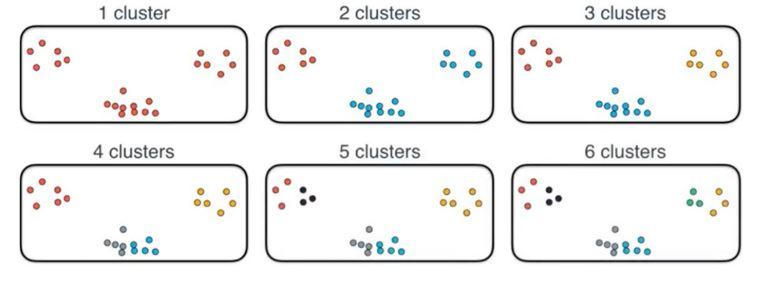2.      Mean-shift

This algorithm attempts to find dense areas of data points by continuously updating the centroids or center points. The updates are based on the mean of all the points in a radius, hence the name mean-shift. Once no more points can be added to the radius, the updates stop. These steps are repeated for many different starting points until the generated clusters are no longer overlapping.

A big advantage of this approach is that we do not need to select the number of clusters. However, one major drawback is that selecting the radius for your potential clusters can be difficult.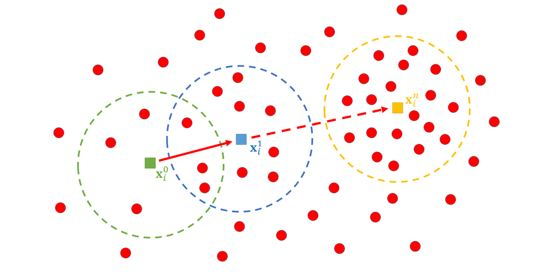3.      Density-Based Spatial Clustering (DBScan)

DBScan is similar with mean-shift in a way that it tries to find areas of high density. It starts by selecting data points, and then looks for nearby neighbors. Neighbors that are close enough are added to the same cluster until no more neighbors are found. Un-assigned data points will then be selected to repeat the same process to create a new cluster.

One major advantage of this method is that it leaves outliers not clustered, whereas the previous algorithms mentioned would have every data point clustered. The downside is that we need to select the maximum distance measure for points to be considered neighbors, which may still vary between various clusters.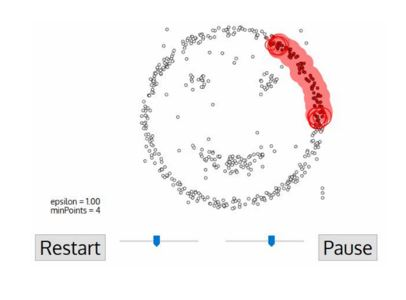4.      Gaussian Mixture Models (GMM)

GMM is sometimes viewed as extension to the ideas behind K-means. However, it tries to describe clusters not only by their means or centroids, but also by the distribution around the means. It calculates the probability that each point belongs to a cluster, and then tries to move the centroids around to maximize the sum of those probabilities.

One huge advantage of this approach is that clusters can have any shape, and that each data point can belong to multiple clusters with varying likelihood.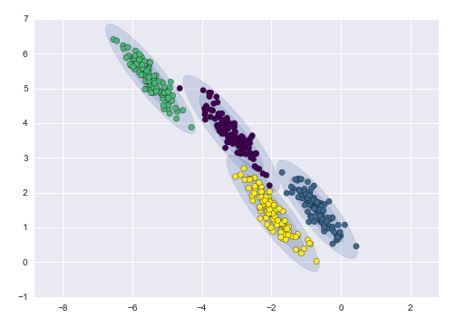Hierarchical Clustering

Unlike partitional clustering, this type of clustering is well suited for hierarchical data such as taxonomies. It creates a tree of clusters that are generated by either:

·        Dividing the full dataset into smaller and smaller clusters (Divisive or ‘top-down’)

·        Grouping individual data points into larger and larger clusters (Agglomerative or ‘bottom-up’)

Nested clusters can be visualized using a tree referred to as a dendrogram.Bayesian Clustering

This type of clustering assumes that data are composed of distributions. As the distance from the distribution center increases, the probability that a point belongs to that distribution decreases. In the image below, the more transparent bands around each cluster show that decrease in probability.Conclusion

Depending on the type of data, these algorithms will perform differently. Therefore, it is very important to know which algorithms are appropriate for various datasets. Since we are dealing with unlabeled datasets for unsupervised machine learning, it is also up to us to decide what the generated clusters mean and what the algorithm has found. As for most cases in data science, algorithms can only do so much – a value is created when human interface with outputs and create meaning.

Click here to know more about the advanced analytics and data science services by N-PAX. For consultations, you can contact us at this section

Blog Categories